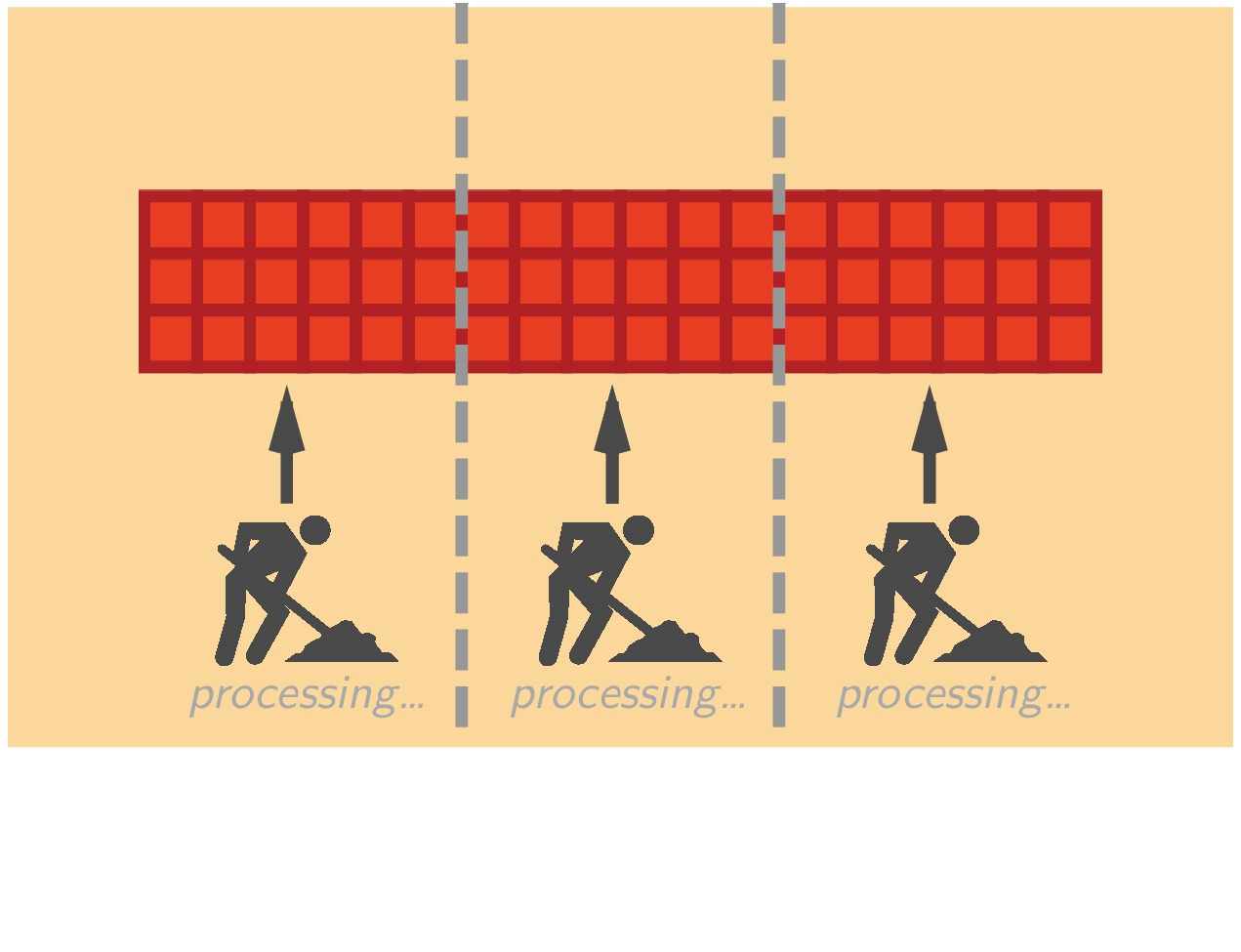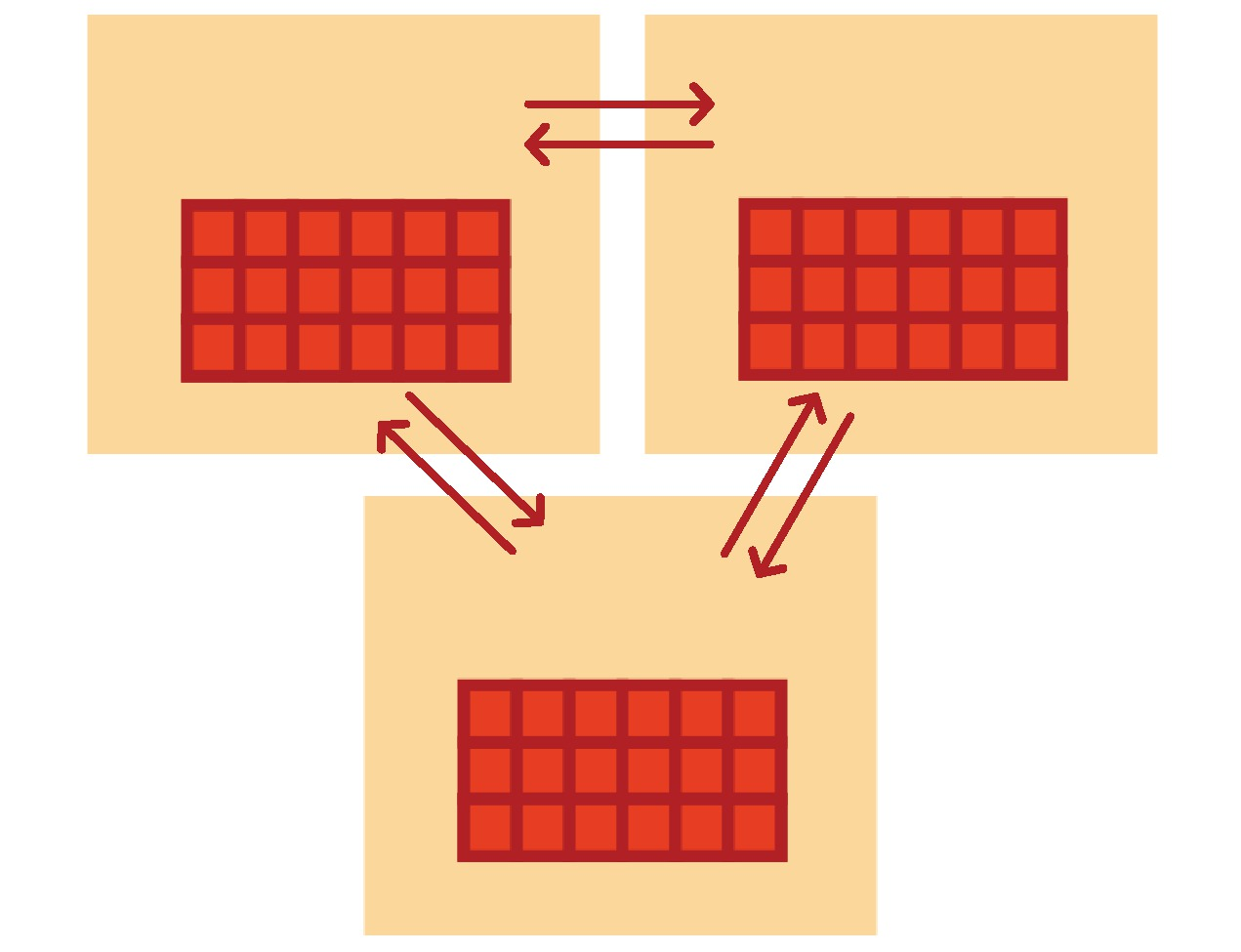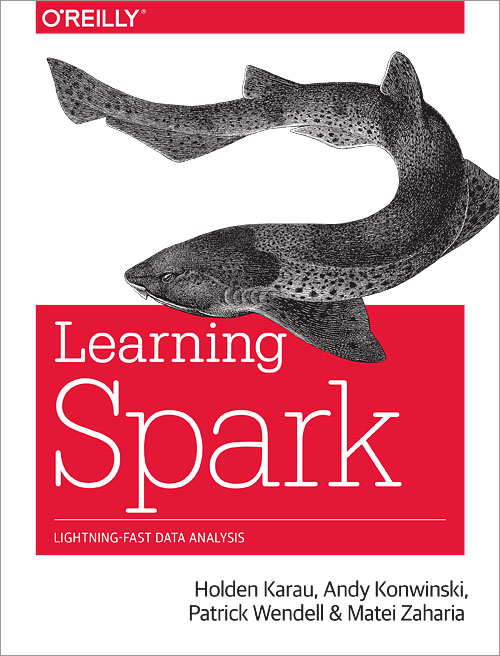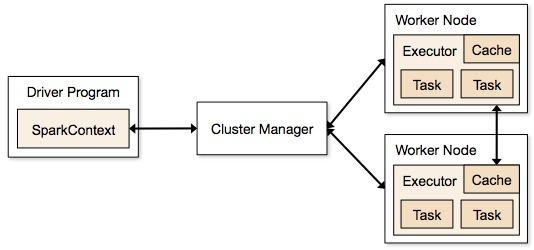# Programmation appliquée en ScalaCopyright © Cay S. Horstmann 2015# Distributed Data-Parallel Programming with Spark

Note: The content of this unit is adapted from the slides of the course "Parallel Programming and Data Analysis"
by Heather Miller at EPFL.

So far we have seen:

• Principles of programming in Scala in sequential and non-distributed style.
• A number of useful tools and technologies based on Scala.
Scala also offers parallel programming via "parallel collections", allowing to use collections in a very similar way that we have learned so far but taking advantage of data parallelism, hence better exploiting multi-core/multi-processor architecture.
Although this aspect will not be treated in the current course.

What we'll see in this course:

• Data parallelism in a distributed setting.
• Distributed collections abstraction from Apache Spark as an
implementation of this paradigm.

# Distribution

Distribution introduces important concerns beyond what we have to
worry about when dealing with parallelism in the shared memory case:

• Partial failure: crash failures of a subset of the machines
involved in a distributed computation.
• Latency: certain operations have a much higher latency than
other operations due to network communication.
Latency cannot be masked completely; it will be an important aspect that also impacts the programming model.

# Data-Parallel to Distributed Data-Parallel

What does distributed data-parallel look like?Shared memory case: Data-parallel programming model. Data partitioned in
memory and operated upon in parallel.

Distributed case: Data-parallel programming model. Data partitioned
between machines, network in between, operated upon in parallel.

# Apache SparkThroughout this part of the course we will use the Apache Spark framework for distributed data-parallel programming.

Spark implements a distributed data parallel model called Resilient Distributed Datasets (RDDs)

# BookLearning Spark by Holden Karau, Andy Konwinski, Patrick Wendell &
Matei Zaharia. O’Reilly, February 2015.

# Resilient Distributed Datasets (RDDs)

RDDs look just like immutable sequential or parallel Scala collections.

 Combinators on Scala parallel/sequential collections: Combinators on RDDs: map map flatMap flatMap filter filter reduce reduce fold fold aggregate aggregate

# Resilient Distributed Datasets (RDDs)

While their signatures differ a bit, their semantics (macroscopically) are the
same:

map[B](f: A => B): List[B] // Scala List
map[B](f: A => B): RDD[B] // Spark RDD

flatMap[B](f: A => TraversableOnce[B]): List[B] // Scala List
flatMap[B](f: A => TraversableOnce[B]): RDD[B] // Spark RDD

filter(pred: A => Boolean): List[A] // Scala List
filter(pred: A => Boolean): RDD[A] // Spark RDD


# Resilient Distributed Datasets (RDDs)

While their signatures differ a bit, their semantics (macroscopically) are the
same:

reduce(op: (A, A) => A): A // Scala List
reduce(op: (A, A) => A): A // Spark RDD

fold(z: A)(op: (A, A) => A): A // Scala List
fold(z: A)(op: (A, A) => A): A // Spark RDD

aggregate[B](z: => B)(seqop: (B, A) => B, combop: (B, B) => B): B // Scala
aggregate[B](z: B)(seqop: (B, A) => B, combop: (B, B) => B): B // Spark RDD


# Resilient Distributed Datasets (RDDs)

Using RDDs in Spark feels a lot like normal Scala sequential/parallel
collections, with the added knowledge that your data is distributed across
several machines.

Example:

Given, val encyclopedia: RDD[String], say we want to search
all of encyclopedia for mentions of HEIG-VD, and count the number of pages that
mention HEIG-VD.

val result = encyclopedia.filter(page => page.contains("HEIG-VD"))
.count()


# Example: Word Count

The “Hello, World!” of programming with large-scale data.

// Create an RDD
val rdd = spark.textFile("hdfs://...")

val count = ???


# Example: Word Count

The “Hello, World!” of programming with large-scale data.

// Create an RDD
val rdd = spark.textFile("hdfs://...")

val count = rdd.flatMap(line => line.split(" ")) // separate lines into words


# Example: Word Count

The “Hello, World!” of programming with large-scale data.

// Create an RDD
val rdd = spark.textFile("hdfs://...")

val count = rdd.flatMap(line => line.split(" ")) // separate lines into words
.map(word => (word, 1))           // include something to count


# Example: Word Count

The “Hello, World!” of programming with large-scale data.

// Create an RDD
val rdd = spark.textFile("hdfs://...")

val count = rdd.flatMap(line => line.split(" ")) // separate lines into words
.map(word => (word, 1))           // include something to count
.reduceByKey(_ + _)               // sum up the 1s in the pairs


That’s it.

# Transformations and Actions

Collection methodes that you have already seen in the Scala standard library
are divided into two major groupes:

Transformers: Return new collections as results. (Not single values.)

Examples: map, filter, flatMap, groupBy

map(f: A => B): Traversable[B] 

Accessors: Return single values as results. (Not collections.)

Examples: reduce, fold, aggregate

reduce(op: (A, A) => A): A

# Transformations and Actions

Similarly, Spark defines transformations and actions on RDDs.

They seem similar to transformers and accessors, but there are some
important differences.

Transformations. Return new RDDs as results.

Actions. Compute a result based on an RDD, and either
returned or saved to an external storage system (e.g., HDFS).

# Transformations and Actions

Transformations are lazy, their result RDD is not immediately computed.

Actions are eager, their result is immediately computed.# Quiz

Consider the following simple example:

val largeList: List[String] = ...
val wordsRdd = sc.parallelize(largeList)
val lengthsRdd = wordsRdd.map(_.length)


What has happened on the cluster at this point?

1. The lengths of the strings in the RDD are calculated on the worker nodes
2. The lengths of the strings in the RDD are calculated on the worker nodes and returned to the master node
3. The sum of the lengths of the strings in the RDD is calculated by the master node
4. Nothing

# Example

Consider the following simple example:

val largeList: List[String] = ...
val wordsRdd = sc.parallelize(largeList)
val lengthsRdd = wordsRdd.map(_.length)
val totalChars = lengthsRdd.reduce(_ + _)


... we can add an action!

# Cluster Topology Matters

If you perform an action on an RDD, on what machine is its result “returned” to?

Example:

val people: RDD[Person] = ...
val first10 = people.take(10)


Where will the Array[Person] representing first10 end up?

# Execution of Spark Programs

A Spark application is run using a set of processes on a cluster. All these processes are
coordinated by the driver program.1. The driver program runs the Spark application, which creates a SparkContext upon start-up.
2. The SparkContext connects to a cluster manager (e.g., Mesos/YARN) which allocates resources.
3. Spark acquires executors on nodes in the cluster, which are processes that run computations and store data for your application.
4. Next, driver program sends your application code to the executors.
5. Finally, SparkContext sends tasks for the executors to run.

# Cluster Topology Matters

If you perform an action on an RDD, on what machine is its result “returned” to?

Example

val people: RDD[Person] = ...
val first10 = people.take(10)


Where will the Array[Person] representing first10 end up?

The driver program.

In general, executing an action involves communication between worker nodes
and the node running the driver program.

# Benefits of Laziness for Large-Scale Data

Spark computes RDDs the first time they are used in an action.

This helps when processing large amounts of data.

Example:

val lastYearsLogs: RDD[String] = ...
val firstLogsWithErrors = lastYearsLogs.filter(_.contains("ERROR")).take(10)


In the above example, lazy execution of filter makes a big difference.

The execution of filter is deferred until the take action is applied.

Spark leverages this by analyzing and optimizing the chain of operations before executing it.

Spark will not compute intermediate RDDs. Instead, as soon as 10 elements of the filtered RDD have been computed, firstLogsWithErrors is done. At this point Spark stops working, saving time and space computing elements of the unused result of filter.

# Caching and Persistence

By default, RDDs are recomputed each time you run an action on them. This can
be expensive (in time) if you need to traverse a dataset more than once.

Spark allows you to control what is cached in memory.

val lastYearsLogs: RDD[String] = ...
val logsWithErrors = lastYearsLogs.filter(_.contains("ERROR")).persist()
val firstLogsWithErrors = logsWithErrors.take(10)


Here, we cache logsWithErrors in memory.

After firstLogsWithErrors is computed, Spark will store the contents of
logsWithErrors for faster access in future operations if we would like to
reuse it.

val numErrors = logsWithErrors.count() // faster


Now, computing the count on logsWithErrors is much faster.

# Other Important RDD Transformations

Beyond the transformer-like combinators you may be familiar with from Scala
collections, RDDs introduce a number of other important transformations.

 sample Sample a fraction of the data, with or without replacement, using a given random number generator seed. union Return a new dataset that contains the union of the elements in the source dataset and the argument. Pseudo-set operations (duplicates remain). intersection Return a new RDD that contains the intersection of elements in the source dataset and the argument. Pseudo-set operations (duplicates remain).

# Other Important RDD Transformations (2)

 distinct Return a new dataset that contains the distinct elements of the source dataset. coalesce Decrease the number of partitions in the RDD to numPartitions. Useful for running operations more efficiently after filtering down a large dataset. repartition Reshuffle the data in the RDD randomly to create either more or fewer partitions and balance it across them. This always shuffles all data over the network.

# Other Important RDD Actions

RDDs also contain other important actions which are useful when dealing with distributed data.

 collect Return all the elements of the dataset as an array at the driver program. This is usually useful after a filter or other operation that returns a sufficiently small subset of the data. count Return the number of elements in the dataset. foreach Run a function func on each element of the dataset. This is usually done for side effects such as interacting with external storage systems. saveAsTextFile Write the elements of the dataset as a text file (or set of text files) in a given directory in the local filesystem, HDFS or any other Hadoop-supported file system. Spark will call toString on each element to convert it to a line of text in the file.

# Pair RDDs

Often when working with distributed data, it’s useful to organize data into
key-value pairs. In Spark, these are Pair RDDs.

Useful because: Pair RDDs allow you to act on each key in parallel or regroup
data across the network.

Spark provides powerful extension methods for RDDs containing pairs (e.g.,
RDD[(K, V)]). Some of the most important extension methods are:

def groupByKey(): RDD[(K, Iterable[V])]
def reduceByKey(func: (V, V) => V): RDD[(K, V)]
def join[W](other: RDD[(K, W)]): RDD[(K, (V, W))]


Depending on the operation, data in an RDD may have to be shuffled among
worker nodes, using worker-worker communication.

This is often the case for many operations Pair RDDs!

# Lab: Spark Setup

First download Spark form here. And then open the archive and goto inside Spark directory and run

./bin/run-example SparkPi 10


It should compute Pi without any error message.

Spark runs on Java 6+ and Python 2.6+. For the Scala API, Spark 1.3.1 uses Scala 2.10. You will need to use a compatible Scala version (2.10.x). The additional information can be found on Spark documentation.

# Part1: Interactive Analysis with the Spark Shell

We use Spark shell to learn the framework. Run following command from Spark home directory:

./bin/spark-shell


We are going to process README.md file of Spark. Run following commands on Spark shell and explain each line and show the results:

scala> val textFile = sc.textFile("README.md")

scala> textFile.count

scala> textFile.first

scala> val linesWithSpark = textFile.filter(line => line.contains("Spark"))

scala> textFile.filter(line => line.contains("Spark")).count


# Part2: Self-Contained Applications

Now we create a very simple Spark application in Scala.

/* SimpleApp.scala */
import org.apache.spark.SparkContext
import org.apache.spark.SparkContext._
import org.apache.spark.SparkConf

object SimpleApp {
def main(args: Array[String]) {
val logFile = "YOUR_SPARK_HOME/README.md" // Should be some file on your system
val conf = new SparkConf().setAppName("Simple Application")
val sc = new SparkContext(conf)
val logData = sc.textFile(logFile, 2).cache()
val numAs = logData.filter(line => line.contains("a")).count()
val numBs = logData.filter(line => line.contains("b")).count()
println("Lines with a: %s, Lines with b: %s".format(numAs, numBs))
}
}


Note that applications should define a main() method instead of extending scala.App. Subclasses of scala.App may not work correctly. What does this program?

Our application depends on the Spark API, so we'll also include an sbt configuration file, build.sbt which explains that Spark is a dependency. This file also adds a repository that Spark depends on:

name := "Simple Project"

version := "1.0"

scalaVersion := "2.10.4"

libraryDependencies += "org.apache.spark" %% "spark-core" % "1.3.1"


For sbt to work correctly, we'll need to layout SimpleApp.scala and build.sbt according to the typical directory structure. Once that is in place, we can create a JAR package containing the application's code, then use the spark-submit script to run our program.

# Your directory layout should look like this
$find . . ./build.sbt ./src ./src/main ./src/main/scala ./src/main/scala/SimpleApp.scala # Package a jar containing your application$ activator package
...
[info] Packaging {..}/{..}/target/scala-2.10/simple-project_2.10-1.0.jar

# Use spark-submit to run your application
\$ YOUR_SPARK_HOME/bin/spark-submit \
--class "SimpleApp" \
--master local \
target/scala-2.10/simple-project_2.10-1.0.jar
...


What is the output of the program?

# Homework: Exploring full-text Wikipedia articles

In this assignment, you will get to know Spark by exploring full-text Wikipedia articles. To start first download the assignment: wikipedia.zip

Gauging how popular a programming language is important for companies judging whether or not they should adopt an emerging programming language. For that reason, industry analyst firm RedMonk has bi-annually computed a ranking of programming language popularity using a variety of data sources, typically from websites like GitHub and StackOverflow. See their top-20 ranking for January 2015 as an example.

In this assignment, we'll use our full-text data from Wikipedia to produce a rudimentary metric of how popular a programming language is, in an effort to see if our Wikipedia-based rankings bear any relation to the popular RedMonk rankings. You'll complete this exercise on just one node (your laptop).

First create a SparkConfig instance. A SparkConfig represents the configuration of your Spark application. It's here that you must specify that you intend to run your application in "local" mode. You must also name your Spark application at this point. Then create a SparkContext. A SparkContext is the "handle" to your cluster. Once you have a SparkContext, you can use it to create and populate RDDs with data. For help, see the Spark API Docs.

# Read-in Wikipedia Data

There are several ways to read data into Spark. The simplest (but most unrealistic) way to read in data is to convert an existing collection in memory to an RDD using the parallelize method of the Spark context.

We have already pre-processed a small chunk of Wikipedia for you, and have made it available in the articles member of the WikipediaData object. Create an RDD (by implementing val wikiRdd) which contains the WikipediaArticle objects of articles.

# Compute a ranking of programming languages

We will use a simple metric for determining the popularity of a programming language: the number of Wikipedia articles that mention the language at least once.

# Rank languages attempt #1: rankLangs

Start by implementing a helper method occurrencesOfLang which computes the number of articles in an RDD of type RDD[WikipediaArticles] that mention the given language at least once.

Computing the ranking, rankLangs using occurrencesOfLang, implement a method rankLangs which computes a list of pairs where the second component of the pair is the number of articles that mention the language (the first component of the pair is the name of the language).

An example of what rankLangs returns might look like this:

List(("Scala",999999),("JavaScript",1278),("LOLCODE",982),("Java",42))


The list should be sorted in descending order. That is, according to this ranking, the pair with the highest second component (the count) should be the first element of the list.

Pay attention to roughly how long it takes to run this part! (It should take tens of seconds.)

How long does the code take? What is the list of ranked languages?

# Rank languages attempt #2: rankLangsUsingIndex

Compute an inverted index: makeIndex

An inverted index is an index data structure storing a mapping from content, such as words or numbers, to a set of documents. In particular, the purpose of an inverted index is to allow fast full text searches. In our use-case, an inverted index would be useful for mapping from the names of programming languages to the collection of Wikipedia articles that mention the name at least once.

To make working with the dataset more efficient and more convenient, implement a method that computes an "inverted index" which maps programming language names to the Wikipedia articles on which they occur at least once.

Implement method makeIndex which returns an RDD of the following type: RDD[(String, Iterable[WikipediaArticle])]. This RDD contains pairs, such that for each language in the given langs list there is at most one pair. Furthermore, the second component of each pair (the Iterable) contains the WikipediaArticles that mention the language at least once.

Hint: You might want to use methods flatMap and groupByKey on RDD for this part.

Computing the ranking: rankLangsUsingIndex

Use the makeIndex method implemented in the previous part to implement a faster method for computing the language ranking.

Like in part 1, rankLangsUsingIndex should compute a list of pairs where the second component of the pair is the number of articles that mention the language (the first component of the pair is the name of the language).

Again, the list should be sorted in descending order. That is, according to this ranking, the pair with the highest second component (the count) should be the first element of the list.

How long does the code take? What is the list of ranked languages?

Can you notice a performance improvement over attempt #1? Why?

# Rank languages attempt #3: rankLangsReduceByKey

In the case where the inverted index from above is only used for computing the ranking and for no other task (full-text search, say), it is more efficient to use the reduceByKey method to compute the ranking directly, without first computing an inverted index. Note that the reduceByKey method is only defined for RDDs containing pairs (each pair is interpreted as a key-value pair).

Implement the rankLangsReduceByKey method, this time computing the ranking without the inverted index, using reduceByKey.

Like in part 1 and 2, rankLangsReduceByKey should compute a list of pairs where the second component of the pair is the number of articles that mention the language (the first component of the pair is the name of the language).

Again, the list should be sorted in descending order. That is, according to this ranking, the pair with the highest second component (the count) should be the first element of the list.

Hint: method mapValues on PairRDD could be useful for this part.

How long does the code take? What is the list of ranked languages?

Can you notice an improvement in performance compared to measuring both the computation of the index and the computation of the ranking as we did in attempt #2? If so, can you think of a reason?